Summerschool Mathematical Modelling, Nonlinear Dynamics, Stochastic and Complex Systems
August 19-25, 2018

Content

 This summer school will give a unique introduction into modelling with differential equations combined with data analysis. This includes both deterministic dynamical systems theory as well as stochastic systems. Lectures will cover mathematical techniques for analysing complex systems of various fields in science and engineering. All theoretical parts of the course will be accompanied with hands on exercises using real life examples ranging from mechanics over medicine to economy.

 Mathematical modelling, differential equations, existence and stability theory Numerical methods Theory of invariant manifolds Periodic solutions Bifurcations and the implicit function theorem Time series analysis Stochastic differential equations Travelling waves and pattern formation

Mathematical modelling, differential equations, existence and stability theory

 Important examples of differential equations in science and engineering, mathematical modelling, elementary solution methods, existence and uniqueness, numerical solutions, phase space, Lyapunov stability, asymptotic stability and Lyapunov functions. Practical exercise: Numerical solution of a differential equation with the Picard iteration. Infection modelling and stability analysis of the model.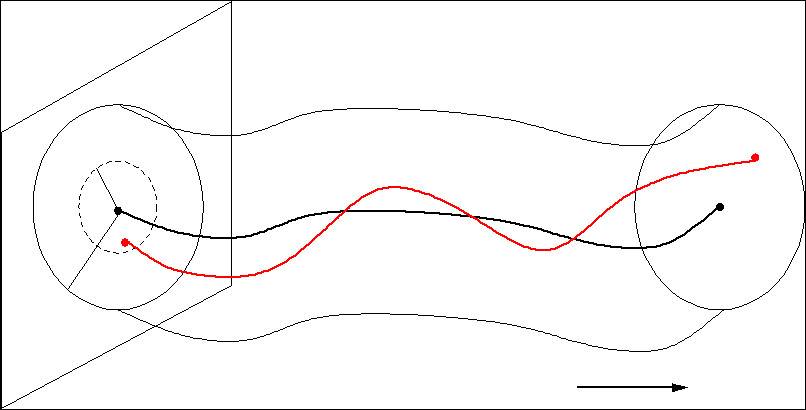Lyapunov stability Top

Numerical methods
 Runge-Kutta methods for non-stiff and stiff systems, error estimation, adaptive step size control, sensitivity equations, dynamic optimization, parameter estimation and optimal control. Practical exercise: Optimal control in an artificial pancreas for type 1 diabetics.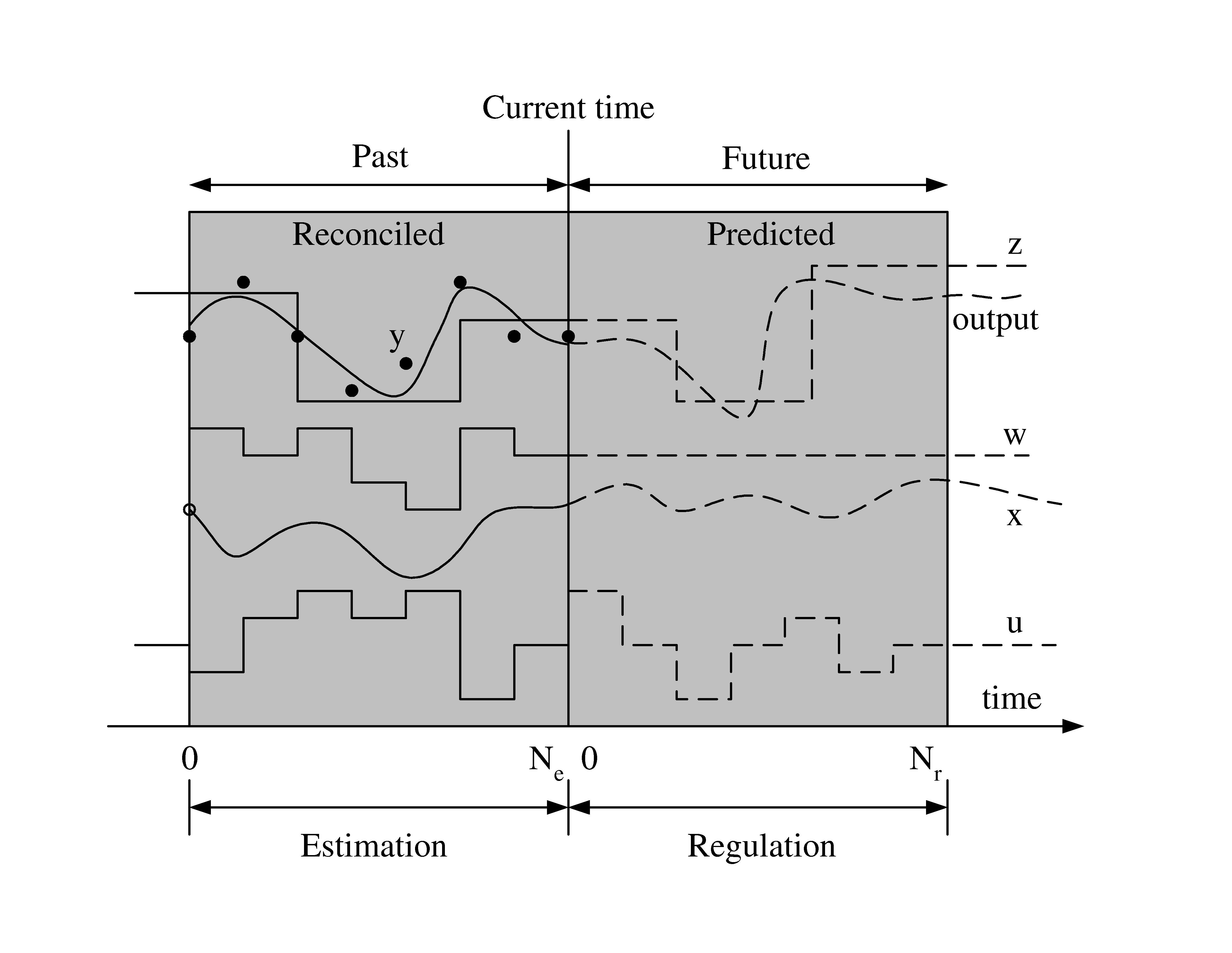Control of glucose level Top

Theory of invariant manifolds
 Stable manifolds, unstable manifolds, center manifolds, homoclinic orbits, heteroclinic orbits and center manifold reduction. Practical exercise: Sensitivity of dependence on initial values in a chemical reaction.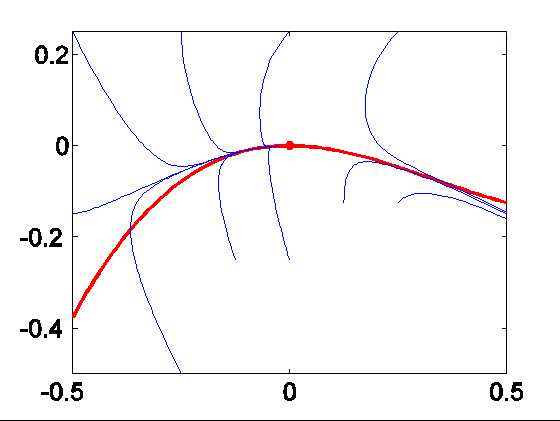Center manifolds reduction Top

Periodic solutions
 Theorem of Poincaré-Bendixon, Poincaré-sections, stability of periodic orbits and forced oscillators. Practical exercise: Modelling a swing; analysis of the Mathieu equation.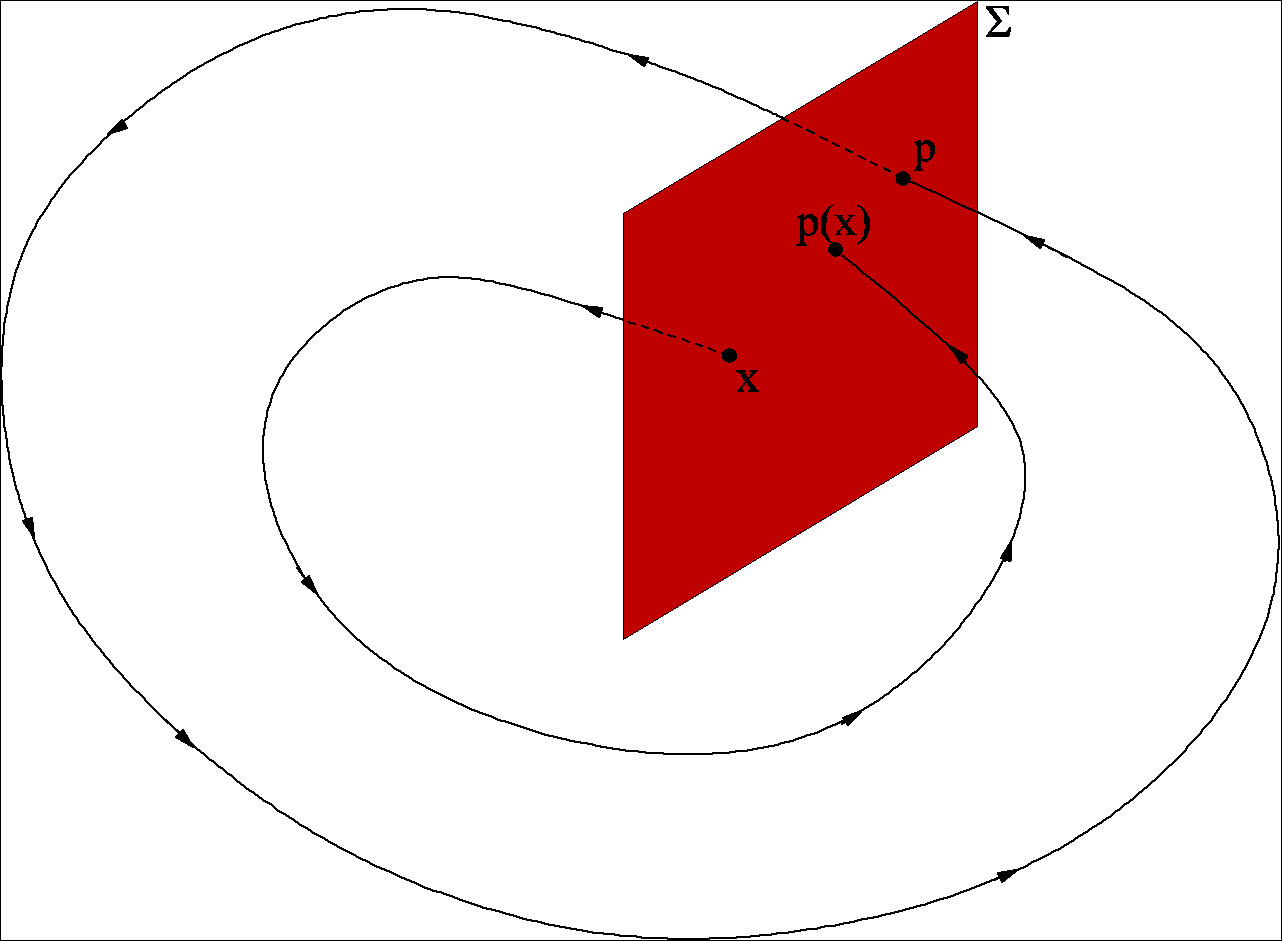Poincaré maps Top

Bifurcations and the implicit function theorem
 Implicit function theorem, structural stability, saddle-node bifurcation, transcritical bifurcation, pitchfork bifurcation, Hopf bifurcation and continuation techniques. Practical exercise: Modelling an electric circuit and Van der Pol oscillator.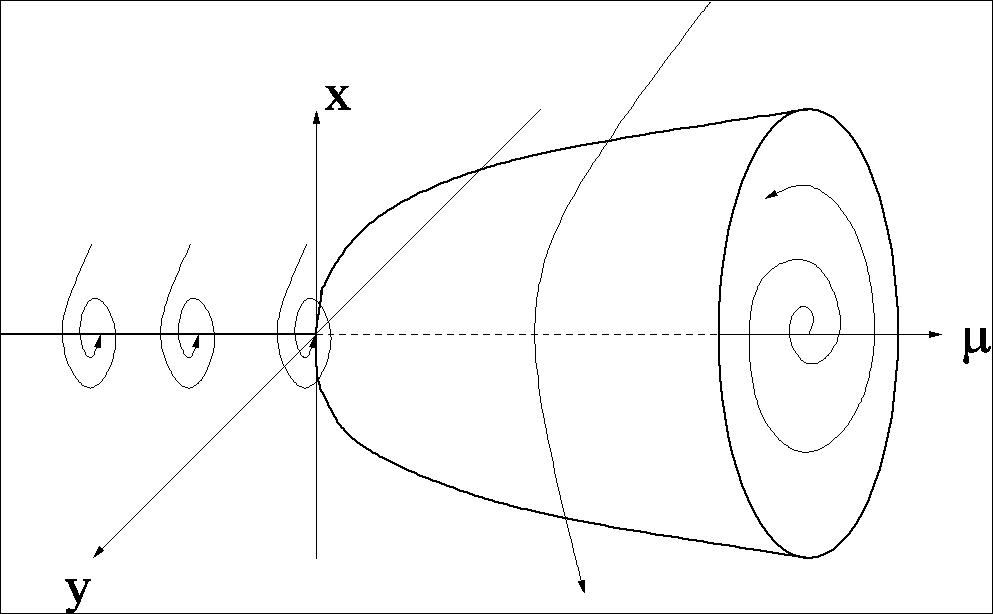Hopf bifurcation Top

Time series analysis
 Characteristics for time series, parametric and nonparametric modelling, models for linear and nonlinear time series, model identification, estimation and verification, predictions in time series. Practical exercise: Prediction of bond prices. Practical exercise: Prediction of bond prices.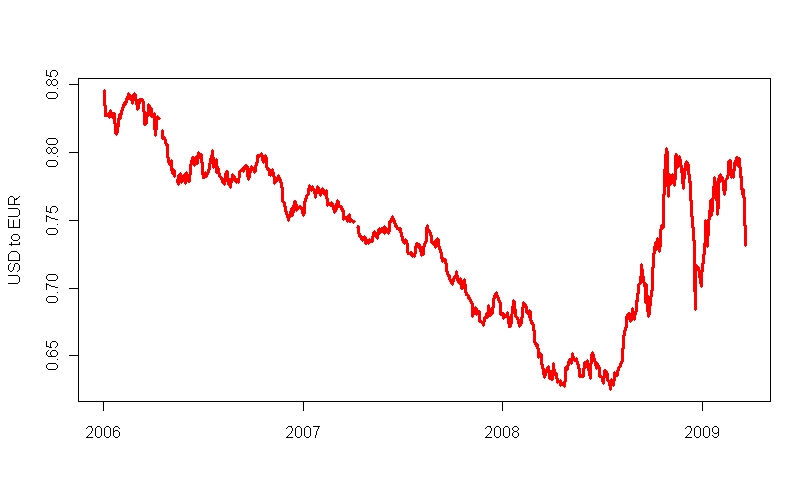Bond prices overtime Top

Stochastic differential equations
 Introduction to stochastic differential equations, Itô and Stratonovich integrals, grey-box modelling, parameter estimation and model building. Practical exercise: Stochastic modelling of the insulin glucose relation.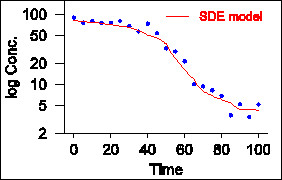Parameter estimation for SDE model Top

Travelling waves and pattern formation
 Nonlinear partial differential equations, travelling waves and soliton solutions, Korteweg de Vries equation, complex pattern formation in reaction diffusion equations, reduction to systems of ordinary differential equations, homoclinic and heteroclinic connections. Practical exercise: Spiral waves in the Belousov-Zhabotinsky reaction.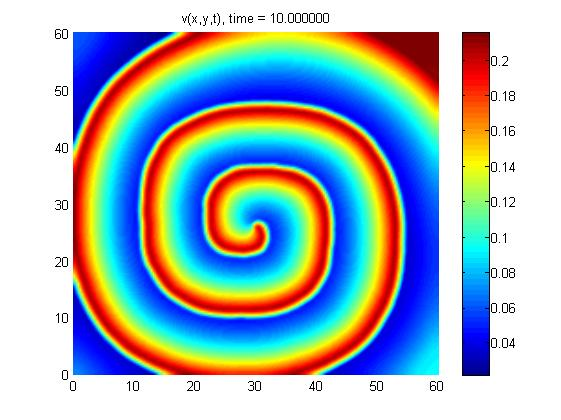Pulse propagation in form of spiral vawes Top

Home   University of Rostock   Institute of Mathematics

created by: Gabriella Keuer
last updated: March 15, 2018